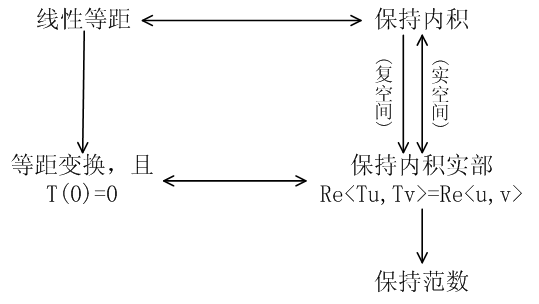# Linear Algebra Done Right第八章注记和部分习题

1 $$\dim\mathrm{null}\,T^k$$ 随着 k 的增加量

$$\dim\mathrm{null}\,T^k$$ 是 k 的单调递增函数，而且当 k 达到某一个数值之后，$$\dim\mathrm{null}\,T^k$$ 就恒定不变了。但是书上并没有说它的增加有何规律，是每次都增加相同的维数，还是增加的维数可能有变化？换句话说，$$\dim\mathrm{null}\,T^{k+1}-\dim\mathrm{null}\,T^k$$ 有什么规律？它是恒定不变的，还是忽大忽小的，或是有什么别的规律没有？

3 引理 8.40 的另一种证明

a) $$(v_1,Nv_1,\dots,N^{m(v_1)}v1,\dots,v_k,Nv_k,\dots,N^{m(v_k)}v_k)$$ 是 $$V$$ 的基底；
b) $$(N^{m(v_1)}v1,\dots,N^{m(v_k)}v_k)$$ 是 $$\mathrm{null}\,N$$ 的基底。

3 设 $$T\in\mathcal L(V)$$，m 是正整数，且 $$v\in V$$ 是满足 $$T^{m-1}v\not=0$$ 但 $$T^mv=0$$ 的向量。证明 $$(v, Tv, T^2v,\dots,T^{m-1}v)$$ 线性无关。

5 设 $$S,T\in\mathcal L(V)$$。证明如果 $$ST$$ 是幂零的，那么 $$TS$$ 也是幂零的。

13 设 $$V$$ 是 n 维复向量空间，且 $$T\in\mathcal L(V)$$ 满足 $$\mathrm{null}\,T^{n-2}\not=\mathrm{null}\,T^{n-1}$$。证明 $$T$$ 至多有两个不同的特征值。

20 设 $$T\in\mathcal L(V)$$ 是可逆变换。证明存在多项式 $$p\in\mathcal P(F)$$ 使得 $$T^{-1}=p(T)$$。

28 设 $$a_0,\dots,a_{n-1}\in\mathbf C$$，某线性变换在标准基底下的矩阵是 $\begin{pmatrix}0&&&&&-a_0 \\ 1&0&&&&-a_1 \\ &1&\ddots&&&-a_2 \\ &&\ddots&&&\vdots \\ &&&&0&-a_{n-2} \\ &&&&1&-a_{n-1}\end{pmatrix}$ 找到它的最小多项式与特征多项式。

30 设 V 是复向量空间，且 $$T\in\mathcal L(V)$$。证明 $$V$$ 不能分解为 $$T$$ 的非平凡不变子空间的直和当且仅当 $$T$$ 的最小多项式形如 $$(T-\lambda I)^{\dim V}$$，其中 $$\lambda\in\mathbf C$$。

|

# Linear Algebra Done Right第七章注记和部分习题

1 命题 7.2 为何在实数空间不成立？

2 $$U$$ 是线性算子 $$T$$ 的不变子空间，那么 $$T^*|_U=(T|_U)^*$$ 是否成立？

$$T=\begin{pmatrix}1&1\\ 0&1\end{pmatrix}$$

$$\forall v, \langle Tv,w\rangle=\langle v,T^*w\rangle$$

$$\forall u\in U, \langle Tu,w\rangle=\langle u,(T|_U)^*w\rangle$$

3 实空间变换矩阵的分块上三角化与实空间的正规、自伴算子谱定理

4 自伴算子乘积什么时候是自伴的？

$$S=\begin{pmatrix}0&1\\ 1&0\end{pmatrix},T=\begin{pmatrix}1&0\\ 0&2\end{pmatrix}$$

$$ST=\begin{pmatrix}0&2\\ 1&0\end{pmatrix}\not=\begin{pmatrix}0&1\\ 2&0\end{pmatrix}=TS$$

$$V=\mathrm{null}\,(T-\lambda_1I)\oplus\mathrm{null}\,(T-\lambda_2I)\oplus\dots\oplus\mathrm{null}\,(T-\lambda_mI)$$

$$\displaystyle TSv=\sum_{i=1}^mTSv_i=\sum_{i=1}^mSTv_i=STv$$

5 正定算子的平方根

$$\begin{pmatrix}\cos\theta&\sin\theta\\ \sin\theta&-\cos\theta\end{pmatrix}$$

$$I$$ 的上述形式的平方根都是自伴的，$$I$$ 也有非自伴的平方根，比如，只要 $$a^2+bc=1$$，那么
$$\begin{pmatrix}a&b\\ c&-a\end{pmatrix}^2=I$$

$$\begin{pmatrix}\lambda_1&0\\ 0&\lambda_2\end{pmatrix}$$

$$\begin{pmatrix}a&b\\ c&d\end{pmatrix}^2=\begin{pmatrix}a^2+bc&b(a+d)\\ c(a+d)&d^2+bc\end{pmatrix}=\begin{pmatrix}\lambda_1&0\\ 0&\lambda_2\end{pmatrix}$$

6 等距变换几个相似概念之间的关系

1) $$T$$ 是等距变换，即 $$\forall u,v\in V,\|Tu-Tv\|=\|u-v\|$$
2) $$T$$ 保持范数，即 $$\forall u\in V,\|Tu\|=\|u\|$$
3) $$T$$ 保持内积，即 $$\forall u,v\in V,\langle Tu,Tv\rangle=\langle u,v\rangle$$$$\|Tku-kTu\|^2=\langle Tku,Tku\rangle-\bar k\langle Tku,Tu\rangle$$$$-k\langle Tu,Tku\rangle+k\bar k\langle Tu,Tu\rangle=0$$

$$\|Tu-Tv\|^2=\|Tu\|^2-2\mathrm{Re}\,\langle Tu,Tv\rangle+\|Tv\|^2$$
$$\|u-v\|^2=\|u\|^2-2\mathrm{Re}\,\langle u,v\rangle+\|v\|^2$$

 侯维民. 关于正交变换两种定义方式的探讨 [J]. 高等数学研究, 2005, 8(1): 44-45.
 [美] Peter.D.Lax. 线性代数及其应用 [M]. 傅莺莺，沈复兴，译. 北京：人民邮电出版社，2009.

7 极分解的另一种形式

5 证明如果 $$\dim V\ge 2$$，那么所有 $$V$$ 上的正规算子的集合不是 $$\mathcal L(V)$$ 的子空间。

$$\begin{pmatrix}2&1\\ -1&2\end{pmatrix}+\begin{pmatrix}2&1\\ 1&2\end{pmatrix}=\begin{pmatrix}4&2\\ 0&4\end{pmatrix}$$

7 证明如果 $$T\in\mathcal L(V)$$ 是正规算子，那么对所有正整数 k，有 $$\mathrm{null}\,T^k=\mathrm{null}\,T, \mathrm{range}\,T^k=\mathrm{range}\,T$$。

14 设 $$T\in\mathcal L(V)$$ 是自伴算子，$$\lambda\in\mathbf F, \epsilon>0$$，证明如果存在向量 $$v\in V$$ 满足 $$\|v\|=1$$ 且 $$\|Tv-\lambda v\|<\epsilon$$，那么 $$T$$ 有特征值 $$\lambda’$$ 满足 $$|\lambda-\lambda’|<\epsilon$$。

$$\min_i |\lambda_i-\lambda|^2\le\sum_{i=1}^n|\lambda_i-\lambda|^2v_i^2=\|Tv-\lambda v\|^2<\epsilon^2$$

18 证明如果 $$T$$ 是 $$V$$ 上的半正定算子，那么对任意正整数 k，$$T^k$$ 也是半正定的。

$$\langle T^kv,v\rangle=\langle T^{\frac{k}{2}}v,T^{\frac{k}{2}}v\rangle\ge 0$$

$$\langle T^kv,v\rangle=\langle TT^{\frac{k-1}{2}}v,T^{\frac{k-1}{2}}v\rangle\ge 0$$

24 设 $$T\in\mathcal L(V)$$，$$S\in\mathcal L(V)$$ 是 V 上的线性等距变换， $$R\in\mathcal L(V)$$ 是 V 上的半正定算子且 $$T=SR$$，证明 $$R=\sqrt{T^*T}$$。

27 证明或给出反例：如果 $$T\in\mathcal L(V)$$，那么 $$T^2$$ 的奇异值是 $$T$$ 的奇异值的平方。

|

# 理解复数域上的向量空间（第一篇）

\begin{aligned}\langle Tu,w\rangle=&\frac{\langle T(u+w),u+w\rangle-\langle T(u-w),u-w\rangle}{4}\\ &+\frac{\langle T(u+iw),u+iw\rangle+\langle T(u-iw),u-iw\rangle}{4}i\end{aligned}

# 理解矩阵与矩阵乘积（二）

$$\varphi(u_1+u_2)=\varphi(u_1)+\varphi(u_2)$$
$$\varphi(ku)=k\varphi(u)$$